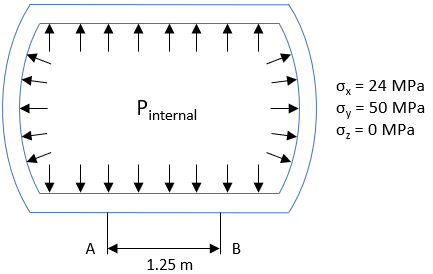## Unpressurized Vessel

The below cross section is of an unpressurized tank vessel with a modulus of elasticity of 200x10^3 MPa, a Poisson’s ratio of 0.25, a coefficient of thermal expansion of 0.000012 m/m°C, and a yield strength of 350 MPa. If the internal pressure were to increase to P such that the wall stresses between Points A & B are below, what is the increased length (along the outer wall) between Points A & B?Hint
For longitudinal strain without a temperature rise:
$$\varepsilon_{x}=\frac{1}{E}[\sigma_x-\nu(\sigma_y+\sigma_z)]$$$where $$E$$ is the modulus of elasticity, $$\nu$$ is Poisson’s ratio, and $$\sigma$$ is the normal stress. Hint 2 Engineering strain: $$\varepsilon=\frac{\Delta L}{L_o}$$$
where $$\Delta L$$ is the change in length of member, and $$L_o$$ is the original member length.
For longitudinal strain without a temperature rise:
$$\varepsilon_{x}=\frac{1}{E}[\sigma_x-\nu(\sigma_y+\sigma_z)]$$$where $$E$$ is the modulus of elasticity, $$\nu$$ is Poisson’s ratio, and $$\sigma$$ is the normal stress. $$\varepsilon_{x}=\frac{1}{200\cdot 10^3MPa}[24MPa-(0.25)(50MPa+0MPa)]$$$
$$=\frac{1}{200\cdot 10^3MPa}[24MPa-12.5MPa]=\frac{11.5}{200\cdot 10^3}=5.75\cdot 10^{-5}$$$For engineering strain: $$\varepsilon=\frac{\Delta L}{L_o}$$$
where $$\Delta L$$ is the change in length of member, and $$L_o$$ is the original member length.
$$5.75\cdot 10^{-5}=\frac{\Delta L}{1,250mm}$$$$$\Delta L =5.75\cdot 10^{-5}(1,250mm)=0.072\:mm$$$
0.072 mm